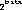`Packages  This Package  Prev  Next  Index  `

# §2.3 Class DirectColorModel

```public  class  java.awt.image.DirectColorModel
extends  java.awt.image.ColorModel  (II-§2.1)
{
// Constructors

// Methods
public final int getAlpha(int  pixel);	§2.3.3
public final int getBlue(int  pixel);	§2.3.5
public final int getGreen(int  pixel);	§2.3.7
public final int getRed(int  pixel);	§2.3.9
public final int getRGB(int  pixel);	§2.3.11
}
```
The direct color model is a color model (II-§2.1) which specifies a translation from pixel values to alpha, red, green, and blue components using the actual bits of the pixel value. This color model is similar to an X11 TrueColor visual.

Many of the methods in this class are final: the underlying native graphics code makes assumptions about the layout and operation of this class and those assumptions are reflected in the implementations of the methods here that are marked final. Applications can subclass this class for other reaons, but they cannot override or modify the behavior of the final methods.

##### DirectColorModel

```public DirectColorModel(int bits, int rmask, int gmask, int bmask) ```
Constructs a direct color model in which each of the given masks specify which bits in the pixels contain the red, green, and blue components.
Pixels described by this color model all have alpha components of 255, indicating that they are fully opaque.
Each of the bit masks must be contigous, and must be smaller than.
Parameters:
```bits - ```the number of bits in a pixel
```rmask - ```the bits in the pixel representing the red component
```gmask - ```the bits in the pixel representing the green component
```bmask - ```the bits in the pixel representing the blue component

### DirectColorModel

```public DirectColorModel(int bits, int rmask, int gmask, int bmask, int amask) ```
Constructs a direct color model in which each of the given masks specify which bits in the pixels contain the alpha, red, green, and blue components.
Each of the bit masks must be contigous, and must be smaller than.
Parameters:
```bits - ```the number of bits in a pixel
```rmask - ```the bits in the pixel representing the red component
```gmask - ```the bits in the pixel representing the green component
```bmask - ```the bits in the pixel representing the blue component
```amask - ```the bits in the pixel representing the alpha component

##### getAlpha

```public final int getAlpha(int pixel) ```
Determines the alpha transparency of a pixel in this color model. The value ranges from 0 to 255. The value 0 indicates that the pixel is completely transparent. The value 255 indicates that the pixel is opaque.
Parameters:
```pixel - ```a pixel value
Returns:
the alpha transparency represented by the pixel value.

```public final int getAlphaMask() ```
Returns:
a mask indicating which bits in a pixel contain the alpha transparency component in this color model.

### getBlue

```public final int getBlue(int pixel) ```
Determines the blue component of a pixel in this color model. The value ranges from 0 to 255. The value 0 indicates no contribution from this primary color. The value 255 indicates the maximum intensity of this color component.
Parameters:
```pixel - ```a pixel value
Returns:
the blue color component represented by the pixel value.
Overrides:
getBlue in class ColorModel (II-§2.1.4).

```public final int getBlueMask() ```
Returns:
a mask indicating which bits in a pixel contain the blue color component in this color model..

### getGreen

```public final int getGreen(int pixel) ```
Determines the green component of a pixel in this color model. The value ranges from 0 to 255. The value 0 indicates no contribution from this primary color. The value 255 indicates the maximum intensity of this color component.
Parameters:
```pixel - ```a pixel value
Returns:
the blue color component represented by the pixel value.
Overrides:
getGreen in class ColorModel (II-§2.1.5).

```public final int getGreenMask() ```
Returns:
a mask indicating which bits in a pixel contain the green color component in this color model.

### getRed

```public final int getRed(int pixel) ```
Determines the red component of a pixel in this color model. The value ranges from 0 to 255. The value 0 indicates no contribution from this primary color. The value 255 indicates the maximum intensity of this color component.
Parameters:
```pixel - ```a pixel value
Returns:
the red color component represented by the pixel value.
Overrides:
getRed in class ColorModel (II-§2.1.7).

```public final int getRedMask() ```
Returns:
a mask indicating which bits in a pixel contain the red color component in this color model.

### getRGB

```public final int getRGB(int pixel) ```
Calculates a single integer representing the alpha, red, green, and blue components of the pixel in this color model. The components are each scaled to be a value between 0 and 255 . The integer returned is the number such that bits 24-31 are the alpha value, 16-23 are the red value, bits 8-15 are the green value, and bits 0-7 are the blue value.
This format is the pixel format of the default RGB colormodel (II-§2.1.9).

Parameters:
```pixel - ```a pixel value
Returns:
an integer representing this color in RGB format.
Overrides:
getRGB in class ColorModel (II-§2.1.8).

`Packages  This Package  Prev  Next  Index`
Java API Document (HTML generated by dkramer on April 22, 1996)